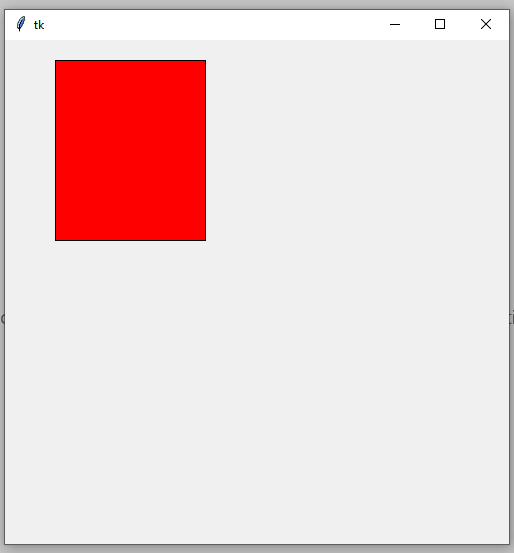# Tkinter Create_rectangle 方法

## 在 Tkinter 中使用 `create_rectangle()` 方法创建矩形

``````from tkinter import *
``````

``````window=Tk()
window.mainloop()
``````

`Canvas` 接受一些参数：`parent``width``height`；在我们的例子中，父窗口对象是一个 `window` 变量。所以基本上，这行代码会在父窗口中创建一个内部窗口。

``````# create an inner window
cv =Canvas(window,width=500,height=500)
cv.pack()
``````

`x0 y0` 参数就像右上角，`x1 y1` 就像右下角。

``````cv.create_rectangle(50,20,200,200,fill="red")
``````

``````from tkinter import *
# create a GUI window widget
window=Tk()
# create an inner window
cv =Canvas(window,width=500,height=500)
cv.pack()
# create a rectangle
cv.create_rectangle(50,20,200,200,fill="red")
window.mainloop()
``````## 在 Tkinter 中创建一个带有文本的矩形

``````# create a red rectangle
cv.create_rectangle(0,0,200,200,fill="red")
# create a blue rectangle
cv.create_rectangle(205,0,405,200,fill="blue")
# create a yellow rectangle
cv.create_rectangle(0,205,200,405,fill="yellow")
# create a green rectangle
cv.create_rectangle(205,205,405,405,fill="green")
``````

``````cv.create_text(100,100,text="Red Rectangle",fill="white")
``````

``````from tkinter import *
# create a GUI window widget
window=Tk()
# create an inner window
cv =Canvas(window,width=500,height=500)
cv.pack()
# create a red rectangle
cv.create_rectangle(0,0,200,200,fill="red")
# This method create a text in rectangle
cv.create_text(100,100,text="Red Rectangle",fill="white")
# create a blue rectangle
cv.create_rectangle(205,0,405,200,fill="blue")
cv.create_text(300,100,text="Blue Rectangle",fill="white")
# create a yellow rectangle
cv.create_rectangle(0,205,200,405,fill="yellow")
cv.create_text(100,300,text="Yellow Rectangle")
# create a green rectangle
cv.create_rectangle(205,205,405,405,fill="green")
cv.create_text(300,300,text="Green Rectangle")
window.mainloop()
``````